# Accounting Equation Notes | Study TS Grewal Solutions - Class 11 Accountancy - Commerce

## Commerce: Accounting Equation Notes | Study TS Grewal Solutions - Class 11 Accountancy - Commerce

The document Accounting Equation Notes | Study TS Grewal Solutions - Class 11 Accountancy - Commerce is a part of the Commerce Course TS Grewal Solutions - Class 11 Accountancy.
All you need of Commerce at this link: Commerce

#### Page No 5.18:

Ques1: What will be effect of the following on the Accounting Equation?
(i) Started business with cash Rs 45,000
(ii) Opened a Bank Account with a deposit of Rs 4,500
(iii) Bought goods from M\s. Sun & Co. for Rs11,200
Ans: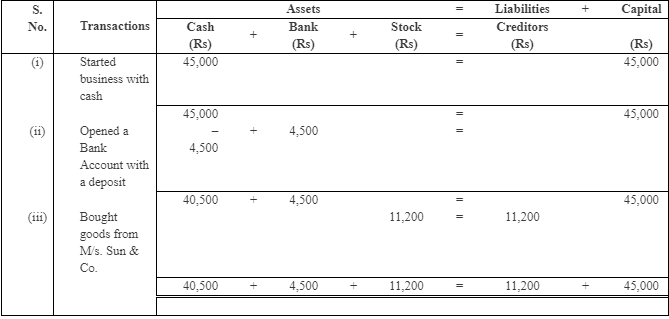Total Assets = Cash + Bank + Stock
= 40,500 + 4,500 + 11,200 = Rs 56,200
Liabilities = 11,200 Capital = 45,000

#### Page No 5.18:

Ques2 :Show the Accounting Equation for the following transactions: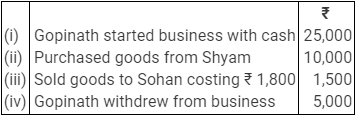Ans: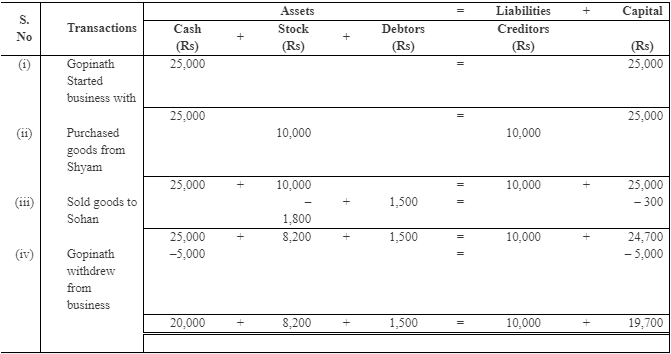Assets=20,000 + 8,200 + 1,500 = Rs29,700
Liabilities=Rs 10,000
Capital=Rs 19,700

#### Page No 5.18:

Ques 3: Show the effect of the following transactions on the Accounting Equation:
(i) Started business with cash Rs 50,000.
(ii) Salaries paid  Rs 2,000.
(iii) Wages Outstanding Rs 200.
(iv) Interest due but not paid Rs 100.
(v) Rent paid in advance Rs 150.

Ans: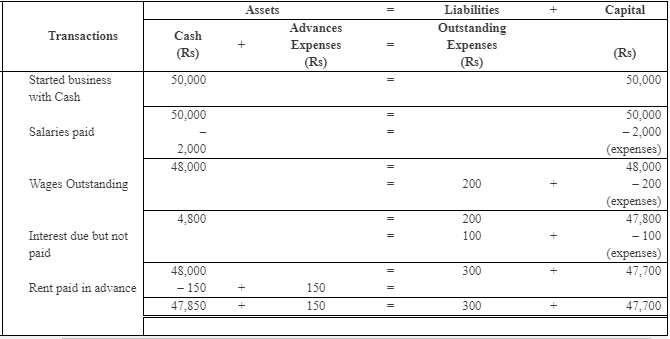Assets = 47,850 + 150 = Rs 48,000
Liabilities = Rs 300
Capital = Rs 47,700

#### Page No 5.18:

Ques 4: What will be the effect of the following on the Accounting Equation?
(i) Harish started business with cash Rs 18,000
(ii) Purchased goods for Cash Rs 5,000 and on credit Rs 2,000
(iii) Sold goods for cash Rs 4,000 (costing Rs 2,400)
(iv) Rent paid Rs 1,000 and rent outstanding Rs 200
Ans: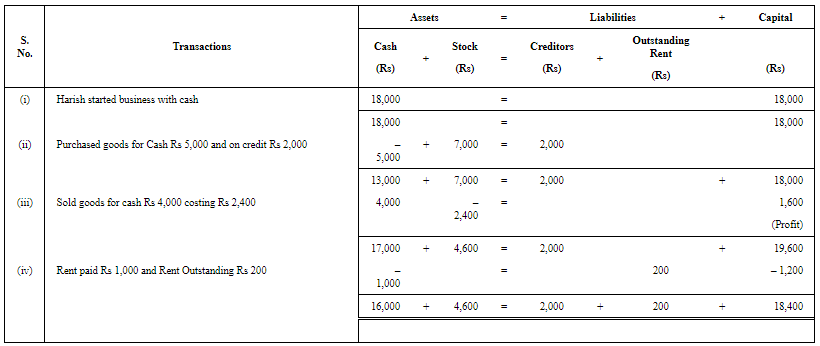Assets = 16,000 + 4,600 = Rs 20,600 Liabilities = 2,000 + 200 = Rs 2,200 Capital = Rs 18,400

#### Page No 5.19:

Ques 5: Prepare Accounting Equation from the following:
(i) Started business with cash Rs 1,00,000 and Goods Rs 20,000.
(ii) Sold goods worth Rs 10,000 for cash Rs 12,000.
(iii) Purchased furniture on credit for Rs 30,000.
Ans: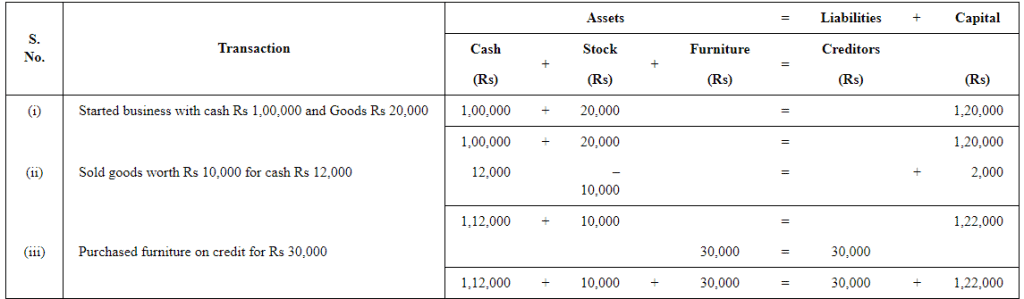Assets = 1,12,000 + 10,000 + 30,000 = Rs 1,52,000
Liabilities = Rs 30,000
Capital = Rs 1,22,000

Ques 6: Prepare an Accounting Equation and Balance Sheet on the following basis:
(i) Ajeet started business with cash Rs 20,000.
(ii) He purchased furniture for Rs 2,000.
(iii) He paid rent of Rs 200.
(iv) He purchase goods on credit Rs 3,000.
(v) He sold goods (cost price Rs 2,000) for Rs 5,000 on cash.
Ans :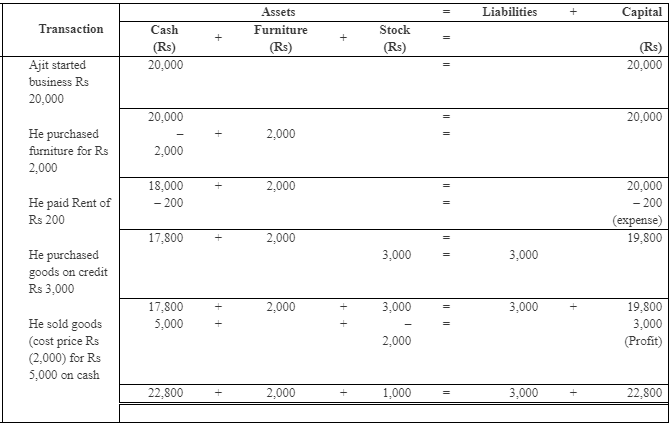Assets = 22,800 + 2,000 + 1,000 = Rs 25,800
Liabilities = Rs 3,000
Capital = Rs 22,800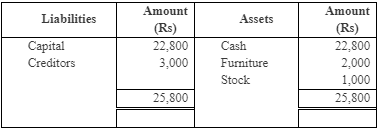#### Page No 5.19:

Ques7 :Prepare an Accounting Equation from the following:
(i) Started business with cash Rs 1,00,000.
(ii) Purchased goods for cash Rs 20,000 and on credit Rs 30,000.
(iii) Sold goods for cash costing Rs 10,000 and on credit costing Rs 15,000 both at a profit of 20%.

Ans: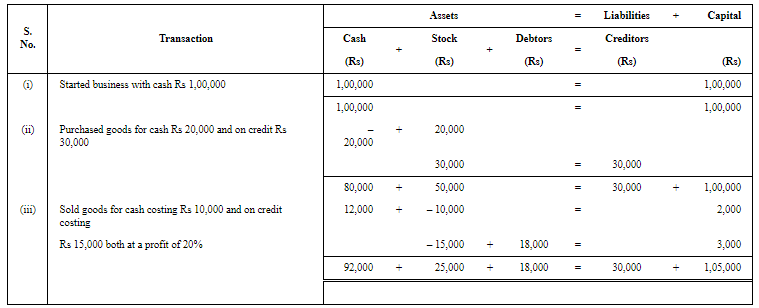Ques 8 : Develop an Accounting Equation from the following transactions: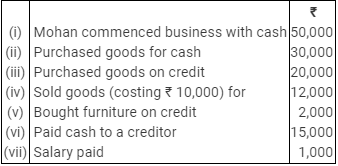Ans: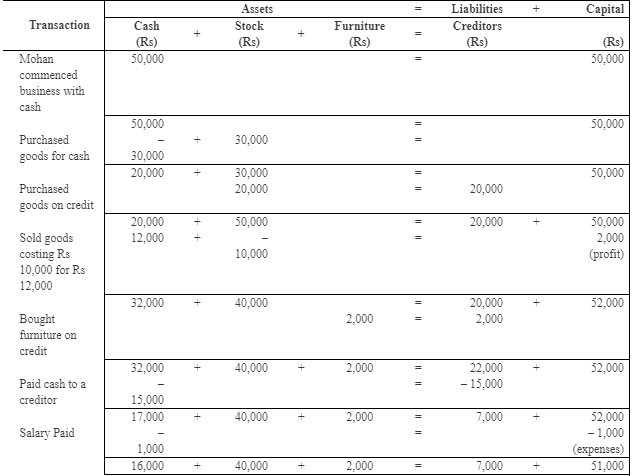Assets = 16,000 + 40,000 + 2,000
= Rs 58,000 Liabilities = Rs 7,000
Capital = Rs 51,000

Ques 9 : Prepare an Accounting Equation on the basis of the following transactions:
(i) Started business with cash Rs 70,000.
(ii) Credit purchase of goods Rs 18,000.
(iii) Payment made to creditors in full settlement Rs 17,500.
(iv) Purchase of machinery for cash Rs 20,000.
(v) Depreciation on machinery Rs 2,000.

Ans: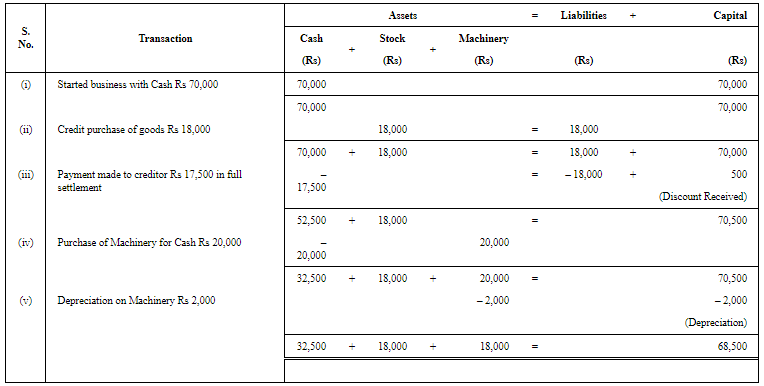Assets = 32,500 + 18,000 + 18,000 = Rs 68,500
Liabilities = NIL
Capital = Rs 68,500

Ques10: Prove that the Accounting Equation is satisfied in all the following transactions of Suresh.
Also prepare a Balance Sheet.
(i) Commenced business with cash Rs 60,000.
(ii) Paid rent in advance Rs 500.
(iii) Purchased goods for cash Rs 30,000 and credit Rs 20,000.
(iv) Sold goods for cash Rs 30,000 costing Rs 20,000.
(v) Paid salary Rs 500 and salary outstanding being Rs 100.
(vi) Bought motorcycle for personal use Rs 5,000.
Ans: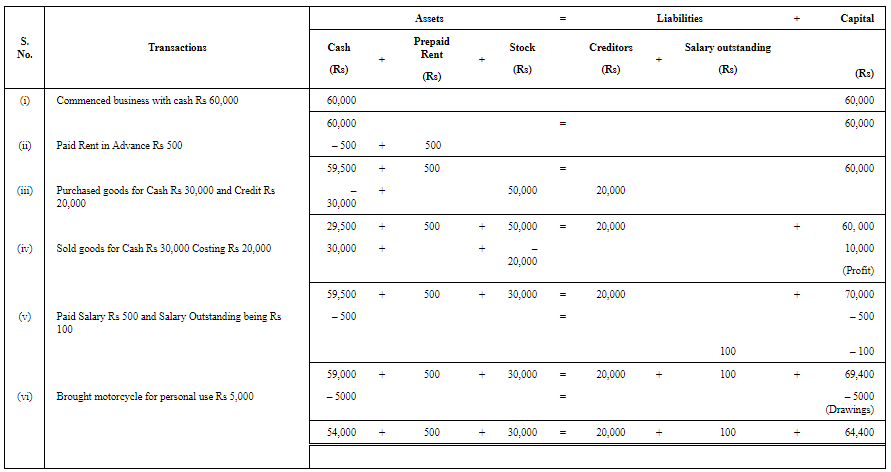Assets = 54,000 + 500 + 30,000 = Rs 84,500
Liabilities = 20,000 + 100 = Rs 20,100
Capital = 64,400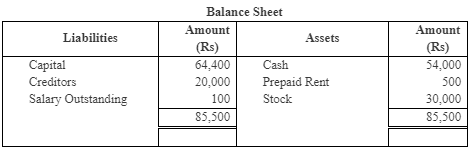#### Page No 5.20:

Ques 11:  Show the effect of the following transactions and also prepare a Balance Sheet:
(i) Started business with cash Rs 60,000.
(iii) Accrued interest Rs 500.
(v) Amount withdrawn Rs 5,000.
Ans: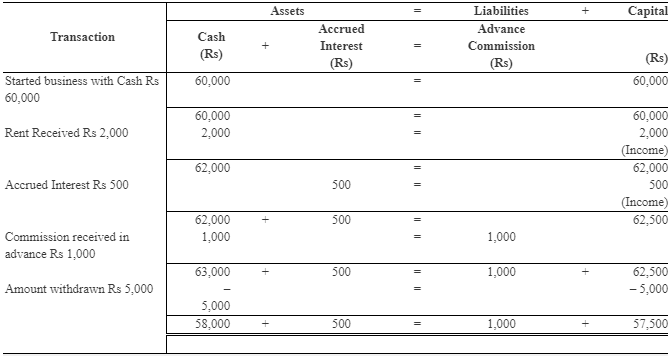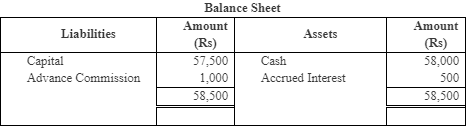Ques12: Prove that the Accounting Equation is satisfied in all the following transactions of Sameer Goel:
(i) Started business with cash Rs 10,000.
(ii) Paid rent in advance Rs 300.
(iii) Purchased goods for cash Rs 5,000 and credit Rs 2,000.
(iv) Sold goods for cash Rs 8,000 costing Rs 4,000.
(v)  Paid salary Rs 450 and salary outstanding being Rs 100.
(vi) Bought motorcycle for personal use Rs 3,000.
Ans: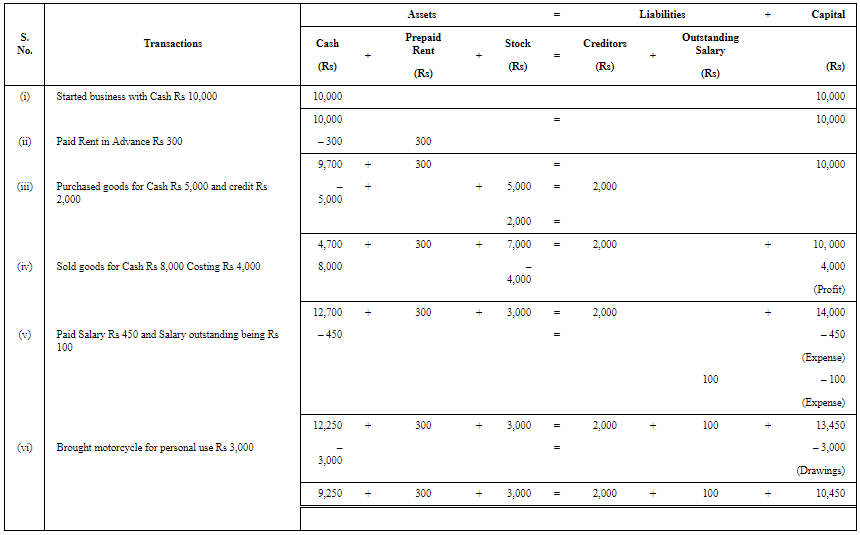Assets = 9,250 + 300 + 3,000 = Rs 12,550
Liabilities = 2,000 + 100 = Rs 2,100
Capital = 10,450

Ques13 : Raghunath had the following transactions in an accounting year:
(i) Commenced business with cash Rs 50,000.
(ii) Paid into bank Rs 10,000.
(iii) Purchased goods for cash Rs 20,000 and credit Rs 30,000.
(iv) Sold goods for cash Rs 40,000 costing Rs 30,000.
(v) Rent paid Rs 500.
(vi) Rent outstanding Rs 100.
(vii) Bought furniture Rs 5,000 on credit.
(viii) Bought refrigerator for personal use Rs 5,000.
(ix) Purchased motorcycle for cash Rs
20,000.
Create an Accounting Equation to show the effect of the above and also show his Balance Sheet.

Ans: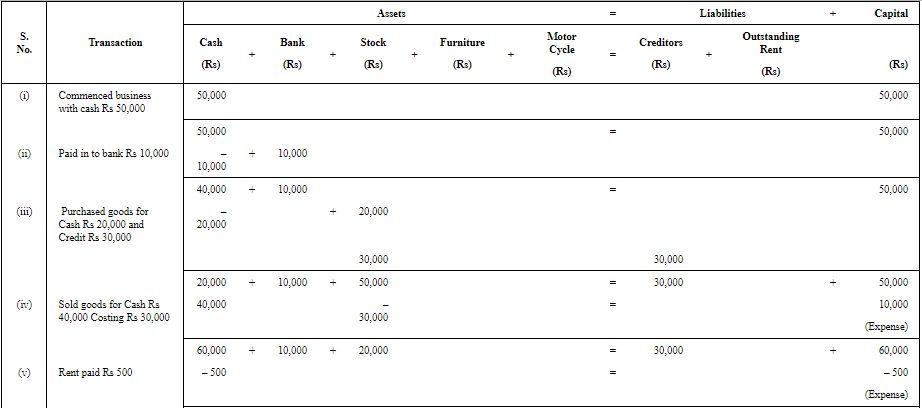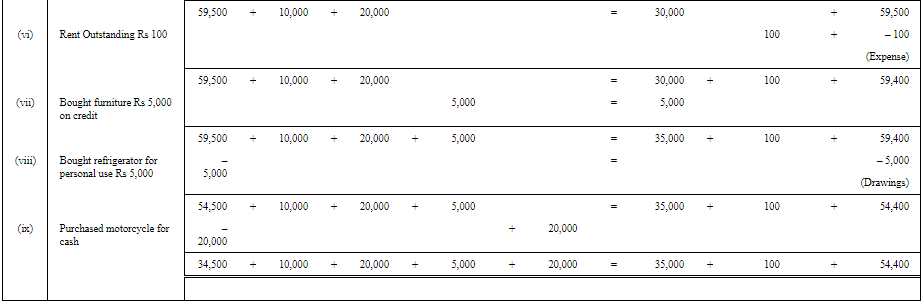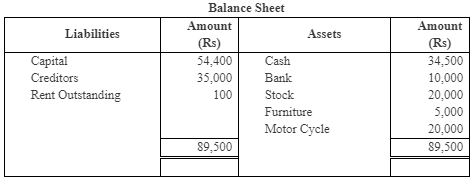Ques 14: Prepare an Accounting Equation from the following:
(i) Started business with cash Rs 50,000 and goods Rs 30,000.
(ii) Purchased goods for cash Rs 30,000 and on credit from Karan Rs 20,000.
(iii) Goods costing Rs 40,000 were sold for Rs 55,000.
(iv) Withdrew cash for personal use Rs 10,000.
(v) Rent outstanding Rs 2,000.
Ans: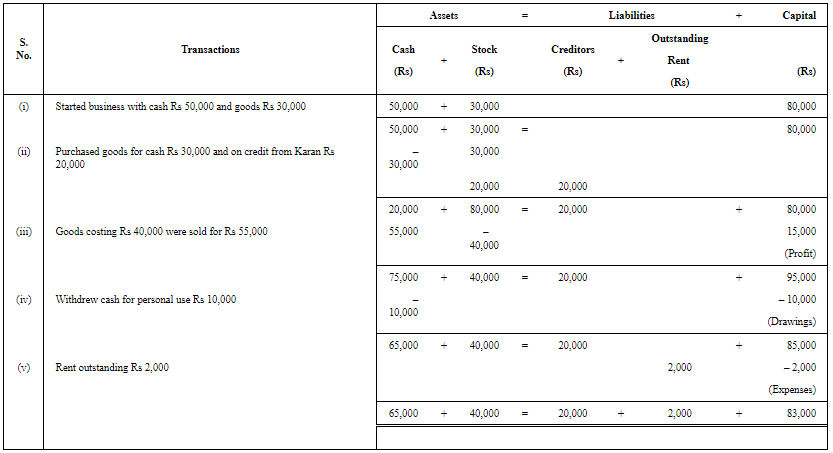#### Page No 5.21:

Ques15 : Show an Accounting Equation for the following transactions:
(i) D. Mahapatra commenced business with cash Rs 50,000 and Rs 1,00,000
by cheque; goods Rs 60,000; machinery Rs1,00,000 and furniture Rs 50,000.
(ii) 1/3rd of above goods sold at a profit of 10% on cost and half of the payment
(iii) Depreciation on machinery provided @ 10%.
(iv) Cash withdrawn for personal use Rs 10,000.
(v) Interest on drawings charged @ 5%.
(vi) Goods Sold to Gupta for Rs 10,000 and received a Bill Receivable for the same amount for 3 months.
(vii) Received Rs 10,000 from Gupta against the Bills Receivable on its maturity.
Ans: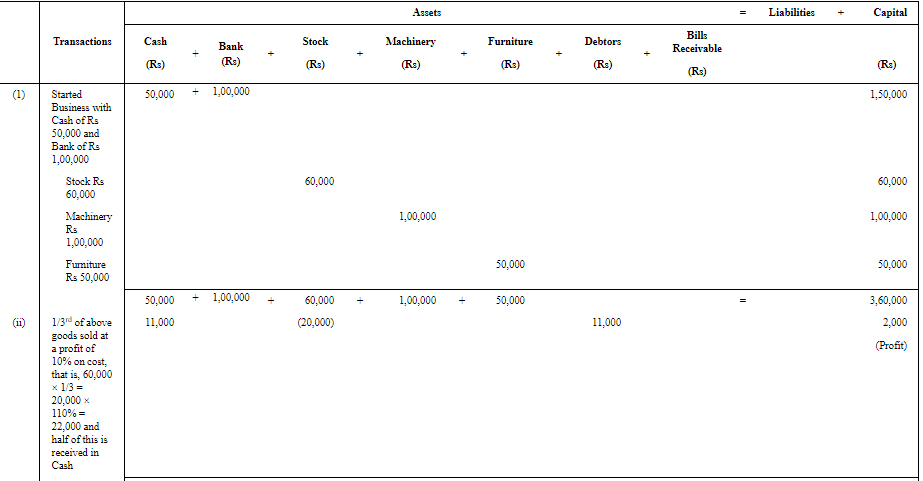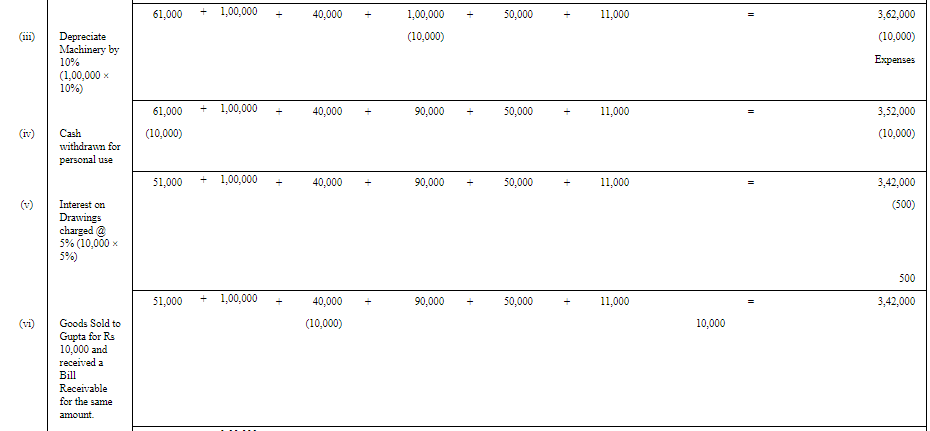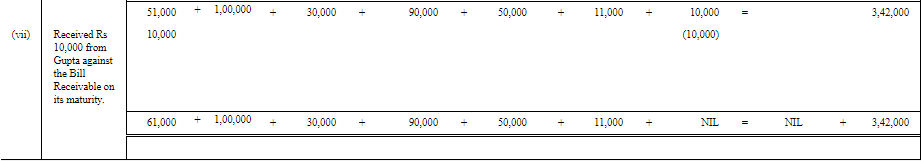#### Page No 5.21:

Ques16: Prepare Accounting Equation from the following:
(a) Started business with cash Rs. 1,00,000.
(b) Purchased goods for cash Rs. 20,000 and on credit Rs. 30,000.
(c) Sold goods for cash costing Rs. 10,000 and on credit costing Rs. 15,000 both at a profit of 20% .
(d) Paid salaries Rs. 8,000.

Ans: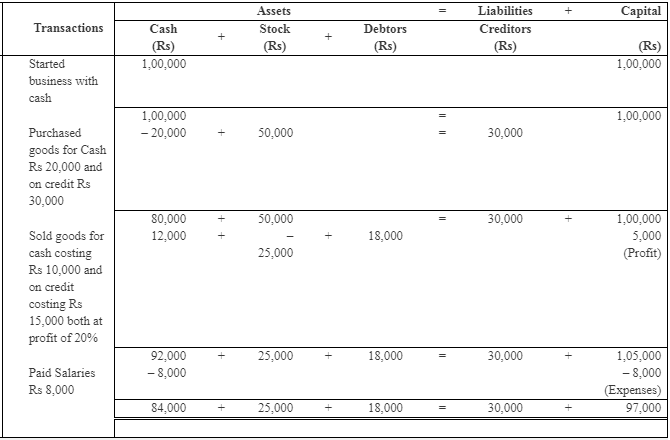Assets = 84,000 + 25,000 + 18,000 = Rs 1,27,000
Liabilities = Rs 30,000
Capital = Rs 97,000

Ques17: Show the accounting equation on the basis of following transactions:
(a) Ram started business with Rs. 25,000.
(b) Purchased goods from Shyam Rs. 10,000.
(c) Sold goods to Sohan costing Rs. 1,500 for Rs. 1,800.

Ans: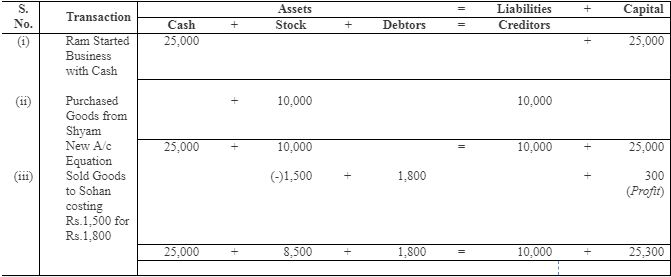#### Page No 5.21:

Ques18: If the capital of a business is Rs. 3,00,000 and liabilities are Rs. 50,000, loss Rs. 70,000, calculate the total assets of the business.
Ans 18: Total Assets = Capital – Loss + Liabilities
= 3,00,000 – 70,000 + 50,000
= Rs 2,80,000

Ques19: If total assets of a business are Rs. 1,30,000 and net worth is Rs. 80,000, calculate the creditors.
Ans: Creditors = Total Assets – Net worth
=1,30,000 – 80,000 =Rs 50,000

Ques20: A commenced his cloth business on 1st April, 2018 with a capital of Rs. 30,000. On 31st March 2019, his assets were worth Rs. 50,000 and liabilities of Rs. 10,000. Find out his closing capital and profits earned during the year.
Ans: Closing Capital = Assets – Liabilities
= 50,000 – 10,000 = Rs 40,000
Profit = Closing Capital – Opening Capital
= 40,000 – 30,000 = Rs 10,000

Ques21: If capital of a business is Rs. 1,40,000 and liabilities are of Rs. 80,000, calculate the total assets of the business.
Ans: Total Assets = Liabilities + Capital
= 80,000 + 1,40,000

= Rs 2,20,000

#### Page No 5.22:

Ques 22: Calculate the total assets if:
(i) Capital is Rs. 40,000.
(ii) Creditors are Rs. 25,000.
(iii) Revenue during the period is Rs. 50,000.
(iv) Expenses during the period are Rs. 40,000.

Ans: Capital after Adjustment of revenue and expenses = Capital + Revenue – Expenses = 40,000 + 50,000 – 40,000 = Rs 50,000
Total Asset = Capital after adjustment + Creditors
= 50,000 + 25,000 = Rs 75,000

Ques23: (a) A had a capital of Rs. 75,000 on 1st April, 2018. He had also goods amounting to Rs. 15,000 which he had purchased on credit and the payment had not been made. Find out the value of the total assets of the business.
(b ) After a period of one month, he came to know that he had suffered a loss of Rs. 1,700. He withdrew Rs. 800 for his personal use. Find out his capital and assets of the business.

Ans: (a) Total Assets = Capital + Creditors for purchase of Goods
= 75,000 + 15,000 = Rs 90,000
(b) Capital at the end = Capital − Loss − Drawings
= 75,000 − 1,700 − 800 = Rs 72,500
Assets = Capital at the end + Creditors for purchase of Goods
= 72,500 + 15,000 = Rs 87,500

Ques24: (a) Mohan started a business on 1st April, 2018 with a capital of Rs. 10,000 and borrowed Rs. 3,000 from a friend. He earned a profit of Rs. 5,000 during the year ended 31st March, 2019 and withdrew cash Rs. 4,000 for personal use. What is his capital on 31st March, 2019? (b) Mahesh started a business with a capital of Rs. 15,000 on 1st April, 2018. During the year, he made a profit of Rs. 3,000. He owes Rs. 2,500 to suppliers of goods. What is the total of assets in his business on 31st March, 2019
Ans: (a) Capital on March 31, 2019 = Capital on April 01, 2018 + Profit − Drawings = 10,000 + 5,000 − 4,000 = Rs 11,000
(b) Total Assets on March 31, 2019 = Capital on April 01, 2018 + Profit + Creditors = 15,000 + 3,000 + 2,500 = Rs 20,500

Ques25: Mohan started a business on 1st April, 2018 with a capital of Rs. 25,000 and a loan of Rs. 12,500 borrowed from Shyam. During 2018-19 he had introduced additional capital of  Rs. 12,500 and had withdrawn Rs. 7,500 for personal use. On 31st March, 2019 his assets were Rs. 75,000. Find out his capital as on 31st March, 2019 and profit made or loss incurred during the year 2018-19.
Ans: Capital on March 31, 2019 = Assets − Loan from Shyam

= 75,000 − 12,500 = Rs 62,500

Profit (or Loss) during the year 2018-19 = Capital on March 31, 2019+ Drawings − (Additional Capital + Capital on April 01, 2018)

= 62,500 + 7,500 − (12,500 + 25,000)
= 70,000 − 37,500 = Rs 32,500

Ques26: On 31st March, 2019, the total assets and external liabilities were Rs. 2,00,000 and Rs. 6,000 respectively. During the year, the proprietor had introduced capital of Rs. 20,000 and withdrawn Rs. 12,000 for personal use. He made a profit of Rs. 20,000 during the year. Calculate the capital as on 1st April, 2018.
Ans: Capital as on March 31, 2019 = Total Assets − External Liabilities
= 2,00,000 − 6,000 = Rs 1,94,000

Capital on April 01, 2018 = Capital on March 31,2019 − Additional Capital + Drawings − Profit
= 1,94,000 − 20,000 + 12,000 − 20,000 = Rs 1,66,000

Ques27: Show an Accounting Equation on the basis of the following transactions: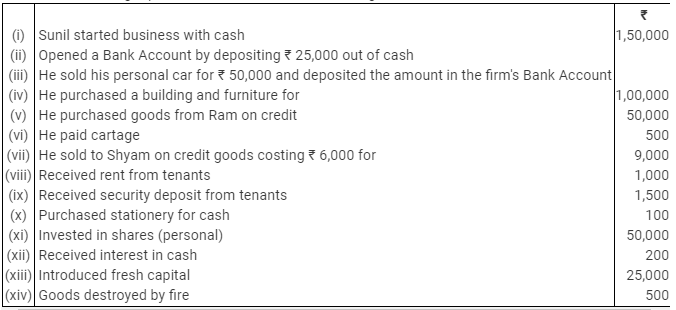Ans: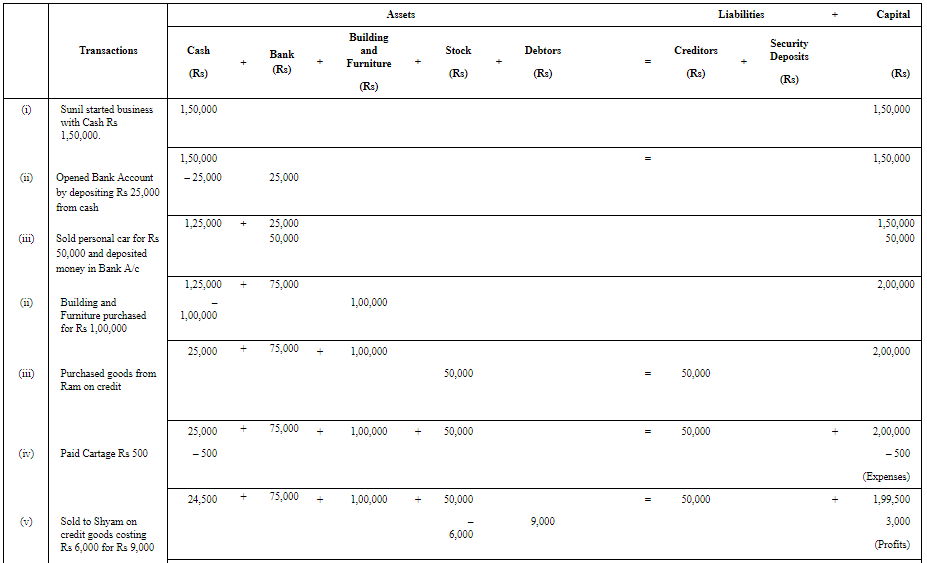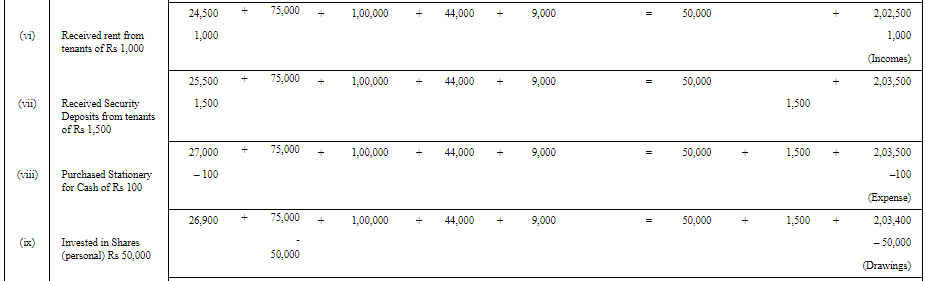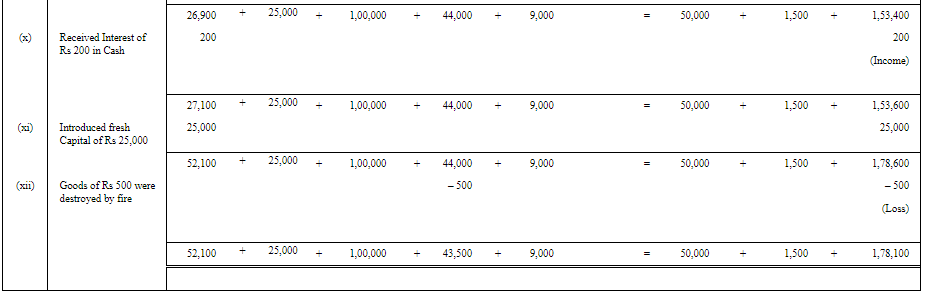Assets = 52,100 + 25,000 + 1,00,000 + 43,500 + 9,000 = 2,29,600
Liabilities = 50,000 + 1,500 = 51,500
Capital = 1,78,100

The document Accounting Equation Notes | Study TS Grewal Solutions - Class 11 Accountancy - Commerce is a part of the Commerce Course TS Grewal Solutions - Class 11 Accountancy.
All you need of Commerce at this link: CommerceUse Code STAYHOME200 and get INR 200 additional OFF

## TS Grewal Solutions - Class 11 Accountancy

48 docs

Track your progress, build streaks, highlight & save important lessons and more!

,

,

,

,

,

,

,

,

,

,

,

,

,

,

,

,

,

,

,

,

,

;